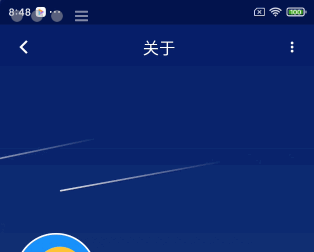### # 前言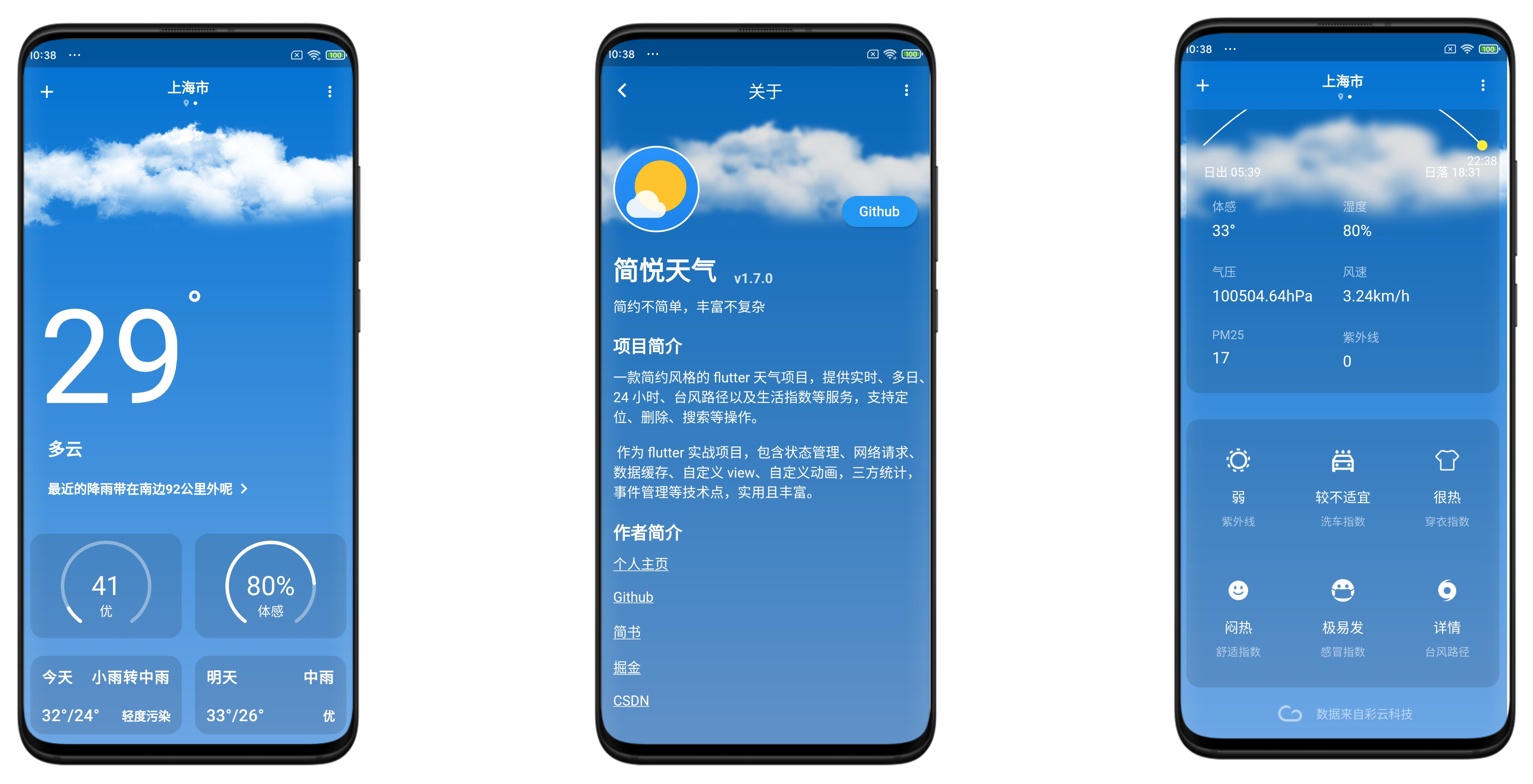• 背景颜色层。从上到下的渐变效果
• 云层。只有一种图片，对其位移、数量、染色做不同变化达到不同效果
• 信息层。包括雨雪、雷暴和晴晚流星效果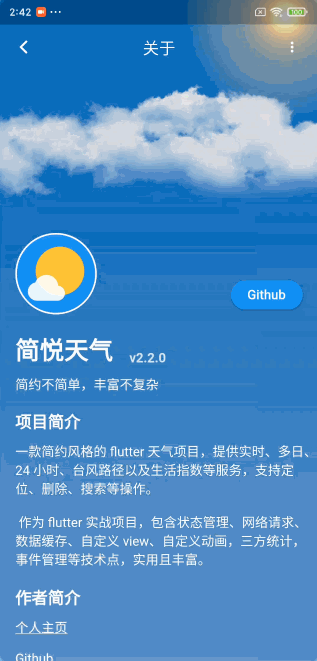• 不断闪烁的星星效果
• 转瞬即逝的流星效果

### # 晴晚

Flutter 同 Android 的绘制有很多相似之处，提供了 canvas 和 paint 类作为画板和画笔，可以绘制基础的图形文字和图片，同样有很多 api 为简单的图形添加渐变、高斯模糊等炫酷的特效 。

#### # 初始化素材和参数

`BlurStyle` 一共有四种类别，分别为：normal、solid、outer和outter，对应下面的效果：`_sigma` 模糊系数越大越模糊，在 normal 下分别设置 0、1、3、7 效果：``````class _StarParam {
double x;
double y;
double alpha = 0.0;
double scale;

_StarParam();

void init() {
alpha = Random().nextDouble();
scale = Random().nextDouble() * 0.1 + 0.6;
x = Random().nextDouble() * 1.wp / scale;
y = Random().nextDouble() * 0.3.hp / scale;
}
}
``````
1
2
3
4
5
6
7
8
9
10
11
12
13
14
15

#### # 绘制

``````  void drawStar(_StarParam param, Canvas canvas) {
if (param == null) {
return;
}
canvas.save();
var identity = ColorFilter.matrix(<double>[
1, 0, 0, 0, 0,
0, 1, 0, 0, 0,
0, 0, 1, 0, 0,
0, 0, 0, param.alpha, 0,
]);
_paint.colorFilter = identity;
canvas.scale(param.scale);
canvas.drawCircle(Offset(param.x, param.y), 3, _paint);
canvas.restore();
}
``````
1
2
3
4
5
6
7
8
9
10
11
12
13
14
15
16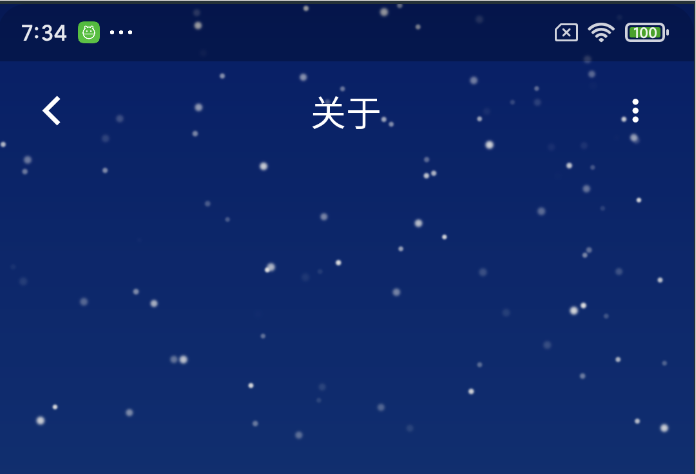#### # 动画

``````  void move() {
if (reverse == true) {
alpha -= 0.01;
if (alpha < 0) {
reset();
}
} else {
alpha += 0.01;
if (alpha > 1.2) {
reverse = true;
}
}
}
``````
1
2
3
4
5
6
7
8
9
10
11
12
13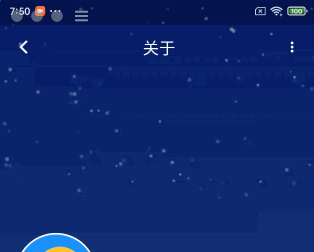### # 流星

#### # 初始化素材和参数

``````  var gradient = ui.Gradient.linear(
const Offset(0, 0),
Offset(_meteorWidth, 0),
<Color>[const Color(0xFFFFFFFF), const Color(0x00FFFFFF)],
);
``````
1
2
3
4
5
6#### # 绘制

``````  void drawMeteor(_MeteorParam param, Canvas canvas) {
canvas.save();
const Offset(0, 0),
Offset(_meteorWidth, 0),
<Color>[const Color(0xFFFFFFFF), const Color(0x00FFFFFF)],
);
canvas.translate(param.translateX, tan(pi * 0.1) *_meteorWidth + param.translateY);
canvas.drawRRect(
RRect.fromLTRBAndCorners(0, 0, _meteorWidth, _meteorHeight,
_meteorPaint);
canvas.restore();
}
``````
1
2
3
4
5
6
7
8
9
10
11
12
13
14
15
16
17
18
19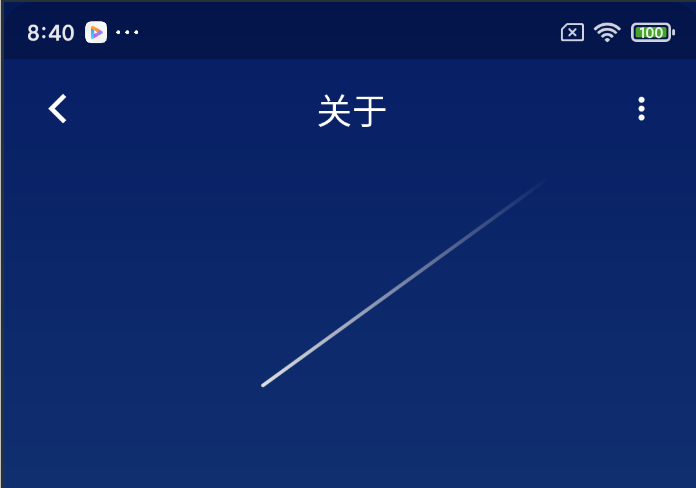#### # 动画

``````class _MeteorParam {
double translateX;
double translateY;
void reset() {
translateX = 1.0.wp + Random().nextDouble() * 20.0.wp;
radians = -Random().nextDouble() * 0.07 - 0.05;
translateY = Random().nextDouble() * 0.5.hp;
}

void move() {
translateX -= 20;
if (translateX <= -1.0.wp) {
reset();
}
}
}
``````
1
2
3
4
5
6
7
8
9
10
11
12
13
14
15
16
17